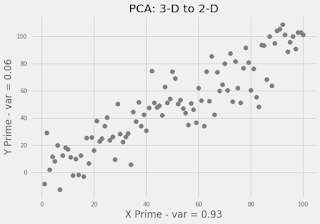## Thursday, March 5, 2020

### Forward

In part one of this series, we took presidential speech data and through the process of Stylometry, we calculated numeric fingerprints for each speech. In this post, we will cover the mathematical process needed to set the stage for visualizations in further posts.

Note: This is an optional post and is very math-heavy. Feel free to skip to post three if you aren't interested in the underlying technology.

### How can we visualize 100 Dimensional Data?

The result of Part One was fingerprints of our speech dataset. These fingerprints cannot easily be visualized as they are high dimensional data. Data is considered high dimensional if its dimensionality is "staggeringly high." In our case, 100 dimensions is very high. Knowing this limitation, it would be handy if there were an easy way of reducing the dimensionality of our data set so we can visualize it. Thankfully we can do this using principal component analysis (PCA).

To understand PCA, let's look at some graphs. Below in figure-1, we can see an animation orbiting 3-D data. From each of these perspectives, the data looks different, but the underlying data never changes.Figure 1 - Orbiting 3D Data
How would we go about projecting this data into 2-D? Each different perspective, we view the 3-D data from results in a different 2-D projection!

Let's apply some real-world intuition. If we were in a room with this cloud of data, we could move around it and shine a flashlight 🔦onto it. The shadow cast from the flashlight is a 2-D projection of our 3-D data. The goal of PCA is to produce the most accurate projection of our data.

The projection is accurate when it preserves as much of the variance as possible. To apply this to our real-word example: We want the shadow cast by our flashlight to be as tall and wide as possible.Figure 2 - Result of our PCA: 2D representation of 3D data
No matter how great our PCA there is always error involved in reducing dimensionality. This error is essential to keep in mind when making significant reductions in dimensionality (like we're doing) as trends in the data may be overlooked.

### The Results

In figure-2, we can see the result of PCA on our 3-D data from figure-1. This projection of our dataset contains the trend visible in the original data and does a good job preserving variance.

### Applying PCA to our fingerprint Data

To apply PCA to our fingerprint data, we utilize the scikit-learn package in python, this gives us an easy way to apply our model to our data. We can provide the function below fingerprint_pca our high dimensional data, and we are returned with the columns of our PCA components along with the error associated with it.

```def fingerprint_pca(data, n_components, fingerprint_col):
"""Return Principal Component Analysis of a fingerprint column.
Fingerprint column is expected to contain a series of list

Keyword arguments:
data -- dataframe containing stylometric fingerprint data
n_components -- number of components to return
fingerprint_col -- column name containing fingerprint data
"""
def _unnester(df, explode):
"""Return a unnested series of columns given a column of nested lists"""
df1 = pd.concat([pd.DataFrame(df[x].tolist(), index=df.index)
.add_prefix(x) for x in [explode]], axis=1)
return df1.join(df.drop(explode, 1), how='left')

df_explode = _unnester(data, fingerprint_col)
pca_model = PCA(n_components).fit(df_explode.iloc[:,0:100].to_numpy())
print(pca_model.explained_variance_ratio_)
pca_res = pca_model.transform(df_explode.iloc[:,0:100].to_numpy())
pca = pd.DataFrame(pca_res,
index=df_explode.uid,
columns=["x", "y"]).reset_index().merge(data, on="uid", how="inner")
return pca

df_pca = fingerprint_pca(data=df, n_components=2, fingerprint_col="fingerprint")
```
Note: The function above is a specific application of PCA to our fingerprint data, for a more generic example reference the API documentation.

At this point It's ok if you don't understand PCA, sometimes I feel like I don't understand it myself. That being said, it's an essential transformation and will be used for visualizations in future posts.